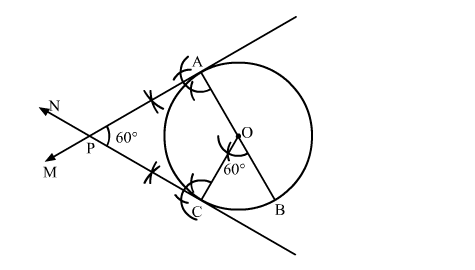# Write the steps of construction for drawing a pair of tangents to a circle of radius 3 cm,`
Question:

Write the steps of construction for drawing a pair of tangents to a circle of radius 3 cm, which are inclined to each other at an angle of 60º.

Solution:

Steps of Construction

Step 1. Draw a circle with centre O and radius 3 cm.

Step 2. Draw any diameter AOB of the circle.

Step 3. Construct ">BOC = 60º such that radius OC cuts the circle at C.

Step 4. Draw AM ⊥ AB and CN ⊥ OC. Suppose AM and CN intersect each other at P.Here, AP and CP are the pair of tangents to the circle inclined to each other at an angle of 60º.